Courses

# Estimation & Valuation 2

## 20 Questions MCQ Test Mock test series of SSC JE Civil Engineering | Estimation & Valuation 2

Description
This mock test of Estimation & Valuation 2 for Civil Engineering (CE) helps you for every Civil Engineering (CE) entrance exam. This contains 20 Multiple Choice Questions for Civil Engineering (CE) Estimation & Valuation 2 (mcq) to study with solutions a complete question bank. The solved questions answers in this Estimation & Valuation 2 quiz give you a good mix of easy questions and tough questions. Civil Engineering (CE) students definitely take this Estimation & Valuation 2 exercise for a better result in the exam. You can find other Estimation & Valuation 2 extra questions, long questions & short questions for Civil Engineering (CE) on EduRev as well by searching above.
QUESTION: 1

### In estimation, deduction for opening with small segmental arches are taken as:

Solution:

For door and windows with small segmental arches (i.e R is very small), the opening is considered as rectangular only.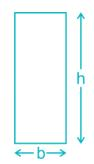Deduction = b × h × thickness of wall

For unit width, b = 1

In Case of segmental arch openings, with rise R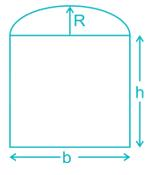Deduction = (b × h × thickness of wall) +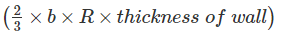For b = 1

Deduction = (1 × h × thickness of wall) +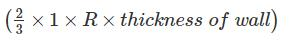QUESTION: 2

### Ashlar stone masonry is measured in:

Solution:

Masonry works are such as brick masonry, concrete block, stone or rubble masonry are generally measured in volume (Example Ashlar masonry is measured in Cubic meter), but are also measured in terms of area where the thickness of masonry is limited to single brick or single block. Example-Tiling is measured in area.

QUESTION: 3

### The quickest method of estimating the building work is:

Solution:

Some Important facts for the different method of estimation are:

1. Centre line method is easy and quick in calculations. In this method sum total length of centre lines of all walls, long and short has to be found out. This method is well suitable for walls of similar cross sections. In this method the total centre line multiplied by breadth and depth of concerned item gives the total quantity of each item.

2. Long wall and short wall method is simple and accurate and there is no chance of any mistake.

QUESTION: 4

Plastering usually “x” mm thick is calculated in square meter. The value of “x” is:

Solution:

The measurement for different works are as follows:

1. Plastering usually 12mm thick is calculated in square meter.

2. Pointing in wall calculated in square meter.

3. Ornamental and large cornice is measured in running meter or running foots.

4. Pillars are taken separately in cubic meter for their net volume.

QUESTION: 5

Consider the following statements:

1. Frames for doors, windows are measured in cubic meters.

2. Shutters for doors, windows are measured in square meters.

The correct statements are:

Solution:

Consider the following points regarding woodwork:

1. Rectangular wooden beams and vertical wooden posts are measured in cubic meters.

2. Frames for doors, windows and ventilators including cupboards and shelves are measured in cubic meters.

3. Shutters for doors, windows and ventilators including cupboards and shelves are measured in square meters.

4. Wooden stairs are measured in terms of number of steps.

5. Wooden cornices and rails are measured in running meters.

QUESTION: 6

The work for the following part of building of specified thickness not measured in square meter:

Solution:

Consider the following points:

1. D.P.C., wall panels, RCC water proof course are measured in square meter.

2. Brick work in arches is measured in cubic meter.

QUESTION: 7

In a particular estimate, work charge establishment charge is taken to be:

Solution:

Work charged establishment is the establishment that is charged to the work directly. During the construction of a building or a project, a certain number of work supervisors, chaukidars, munshies are required to be employed in the project and their salaries are to paid from the work charge establishment of the project. For work charged establishment, 1.5 to 2% of the estimated cost of the project is taken.

QUESTION: 8

Which of the following is not included in Floor area of a building:

Solution:

Floor area of the building is the total area of the floor in between walls and consists of floor of all rooms verandas, corridors etc. Floor area is equal to plinth area minus area occupied by walls.

Following are not included in floor area of the building:

1. Sills of doors and openings are not included in the floor area of the building.

2. Area occupied by walls, pillars and any other intermediate support.

QUESTION: 9

Which of the following method is used for estimation of depreciation of building?

Solution:

Different methods of calculating the depreciation of building are:

1. Straight line method

2. Sinking fund method

3. Quantity survey method

4. Constant percentage method or declining balance method

In Constant percentage method, it is assumed that the property will lose its value by a constant percentage of its value at the beginning of every year.

Annual depreciation: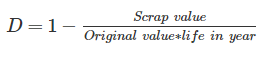QUESTION: 10

Capitalized value of a property is the product of _______.

Solution:

The capitalized value of a property is the amount of money whose annual interest at the highest prevailing rate of interest will be equal to the net income from the property. To determine the capitalized value of a property, it is required to know the net income from the property and the highest prevailing rate of interest.

Therefore, Capitalized Value = Net income x year’s purchase.

QUESTION: 11

Calculate the volume of the embankment (in cubic metre) using trapezoidal method, if the cross-section areas of the three sections of an embankment at an interval of 30 m are 20 square metres, 40 square metres and 50 square metres.

Solution:

Volume of embankment by trapezoidal method is given by:

V = h/2 [a+ a+ 2[a+ a+…. an-1]

h = 30 m

a= 20 m2

a2 = 40 m2

a3 = 50 m2

V = 30/2[20 + 50 + 2

V = 15 

V = 2250 m3

QUESTION: 12

If d be the diameter of MS or tor steel bars in mm, the standard weight (in kg) per metre of the bar is

Solution:

Weight of steel bar = Density x Volume of bar

For 1 meter of length,

Weight of steel bar = Density x Area of Bar x 1

Density of mild steel = 7850 kg per cubic meter

Weight of steel bar= 7850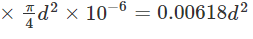QUESTION: 13

A ring type of foundation is constructed with internal and external diameters of 8 m and 12 m respectively. If thickness of the slab is 10 cm and cost of RCC per cubic meter is Rs. 1000, the cost of RCC used in the slab is -

Solution:

Total volume of RCC slab is,V=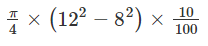Totalcost=V×1000=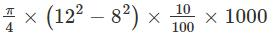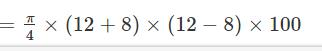QUESTION: 14

A fund which is gradually accumulated and set aside to reconstruct the property after the expiry of its period of utility is known as _________

Solution:

A fund which is gradually accumulated and set aside to reconstruct the property after the expiry of its period of utility is known as sinking fund. The sinking funds may be found out by taking the sinking fund policy with any insurance company or depositing some amount in the bank. Generally while calculating siniking fund, life of the building is considerd. 90% of the cost of construction is used for calculation and 10% is left out as scrap value.

QUESTION: 15

Pillars and painting are computed in:

Solution:

Painting is done on surface area of wall and hence is measured in m2 and pillars are computed in m3 because they occupy volume.

QUESTION: 16

The minimum thickness of load bearing RCC wall is:

Solution:

The minimum thickness of load bearing RCC wall should be 100 mm to prevent failure.

QUESTION: 17

The plinth area includes the area of the balcony which is protected by projection upto:

Solution:

As per IS 3861: 2002, plinth area includes 100 percent area of the balcony protected by projection above and 50 percent area of the unprotected balcony.

QUESTION: 18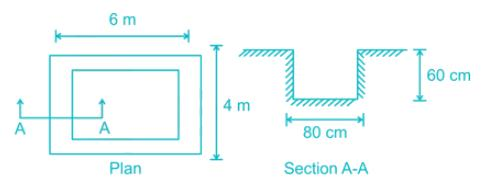The above figure represents plane and section of an excavation layout. The volume of earthwork in excavation of foundation trench is

Solution:

Volume of excavation= perimeter x area

Perimeter= 4+4+2(6- 2x0.8)= 16.8m

Area= 0.8x0.6= 0.48m2; Hence volume= 16.8x0.48= 8.064m3.

QUESTION: 19

The following document contains detailed description of all items of work including their bill of quantities, along with plans and drawings:

Solution:

Tender is an offer to execute some specified work or to supply some specified article at certain rates. While inviting tender the bill of quantities, detailed specifications, conditions of contract and plans and drawings are supplied.

QUESTION: 20

What percentage of the cost of the estimate is provided for the tools and plants?

Solution:

Generally contractor has to arrange for tools and plants. So it is taken to be 1 to 1.5% of estimate cost.